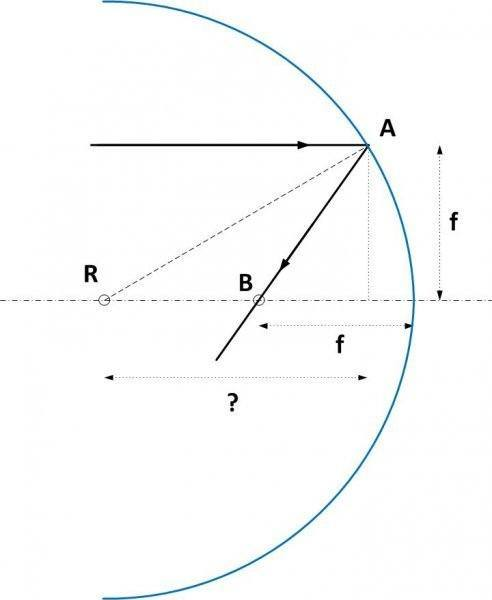# The ratio of the distance of the focus and the point

1. The problem statement: A ray of light is incident on a concave mirror. It is parallel to the principle axis and its height from principle axis is equal to the focal length of the mirror. the ratio of the distance of point B to the distance of the focus from the center of curvature is( AB is the reflected ray)
A)2/√3 B)√3/2 C)2/3 D)1/2

2. Answer to this problem give is: A

## The Attempt at a Solution

: the ray of light is parallel to principle axis so it should pass through the focus. the point of the focus and the point of intersection of the ray and the focus are same. so i thought the answer is 1.
Have I done anything wrong?[/B]

#### Attachments

•pic 1.jpg
6.5 KB · Views: 750

BvU
Homework Helper
What are the relevant equations for a concave mirror ?

radius of curvature is twice that of focal length..

BvU
Homework Helper
So the drawing should not be showing an R where it is drawn now. That's confusing.
What are A and B ?

So the drawing should not be showing an R where it is drawn now. That's confusing.
What are A and B ?
Here that's not R sorry for that .
That's B and the ray that is reflected from the concave mirror is AB

BvU
Homework Helper
Good. Now re-read your first post and explain what ratio is asked for, because it's not really clear what is meant with
the ratio of the distance of point B to the distance of the focus from the center of curvature

•sylwesh98
the ratio = the distance of point b from radius of curvature / the distance from focus to radius of curvature

BvU
Homework Helper
Well, maybe I have to come back on my "Good" in post #6. As you describe it, B = F and the ratio is 1.

Since the answer is given, and it's ##1/{1\over 2}\sqrt 3## I start to suspect that B is not F. Could it be that B is the x-axis coordinate of the point where the beam is reflected on the mirror ?

 oops... see bold faced.

Last edited:
the x-axis coordinate of the point where the beam is reflected on the mirror
B is the point on the principle axis where the reflected ray intersects with the principle axis. A is the point where the ray touches the mirror. (sorry I forgot to mention that point).

is there any relation , if the distance between the ray and principle axis is f? i mean will the ray pass through the focus if the distance between the principle axis and the ray is f, even though the ray is parallel to the axis.

BvU
Homework Helper
That's the idea of the thin lens / mirror rays: what comes in through the focus goes out parallel and vice versa.

My picture so far is (for the B = F)And the one I like is the ? one ...

yah! but the question given is the same as ive mentioned ! i really dont know what he meant to find?

BvU